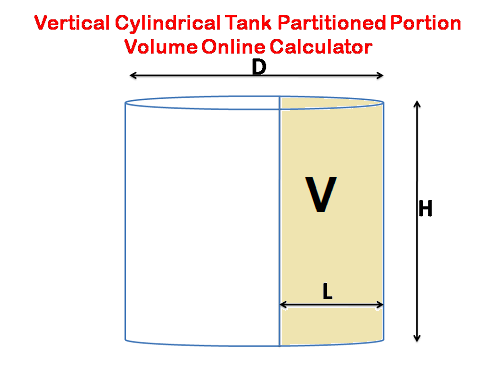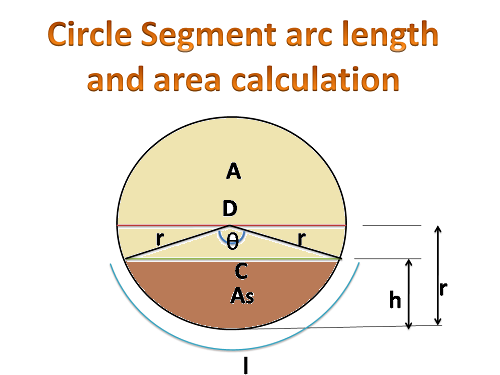# Calculator to Find liquid volume for vertically mounted cylindrical tank partitioned portion

## Formulas with online calculator for vertical cylindrical tank volume and its partitioned section volume.

Sugar industry or other industries using vertically mounded cylindrical tanks for sealing of vacuum equipment like vacuum condensers and multiple effect evaporator lost body liquid extraction (outlet). Sometimes it has also called mound.

In this cylindrical tank sometimes inside of the tank might be separated by vertical plate for easily operation purpose. While providing the partition in this single tank we can use both purposes sealing as well as extraction (withdrawal).

If we using the single mound for sealing and extraction purpose than we must find the volume of the sealing portion for maintain the vacuum in system.

The partition plate provided in exactly center of the tank than it was easy to finding the volume by the formula ( π r2h / 2). But the partition plate is having more or less than the center portion than we can calculate volume by the following formulas.### Data required for  calculation :

Dia of the Cylinder tank = D (mtrs)

Vertical height of the cylindrical tank  =  H  mtrs ( Height should be take upto overflow level of the partitioned plate for sealing mounds)

Perpendicular length from inside partition plate to tank edge =  L in mtrs

### Formulas for calculations:

In this calculation first part is to be find the bottom area of the partitioned portion of the tank.

i.e bottom of the tank having circular shape so we should find the segment of the circle area than its area to be multiply with the height of the tank.Radius of tank (cylinder) = r = D/2

Here to be check radius of the tank is less than or greater than of the L (Perpendicular length from inside partition plate to tank edge).

If the  L > r than take the value ( D – L ) to be take instead of L valve.

Angle   =  θ2 cos -1 ((r – L )/ r  )

Chord length of the circle = = c = 2 √ h (2r – L )

Arc  Length of the circle segment   =  l  = 0.01745 x r x θ

Area of the segment = As = 1/2 (rl – c( r – L))

Circle area except segment = Ar = π r 2  – As

Volume of the liquid in the thank = A x H  ( Here  L < r than take A = As and for L > r than take A = Ac )

### Online Calculator for find liquid volume in cylindrical tank

Related Topics

Sugar Industry Equipment Design and Drawing Online Calculations.

Formulas and design consideration parameters in Robert Evaporator Design

Formulas for Sugar Industry boiling house stock calculation.

NPSH calculation,NPSHa,NPSHr formulas, Pump cavitation , Pump suction and delivery lines head loss with online calculator.

Formula for evaluating Horizontal Cylinder volume at different levels.

Hi friends Thanks for reading and using our online calculator. I Hope you liked it.  Give feed back, comments and please don’t forget to share it.